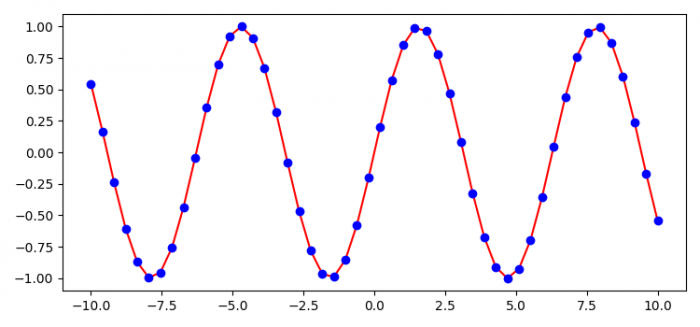# How to plot a line (polygonal chain) with matplotlib with minimal smoothing?

To plot a line (polygonal chain) with matplotlib with minimal smoothing, we can take the following steps −

• Set the figure size and adjust the padding between and around the subplots.
• Initalize a variable, N, to get the number of data points.
• Create x and y data points using numpy.
• Get 1-D monotonic cubic interpolation, using pchip() method.
• Plot (x, interp(x)) and (x, y) data points using numpy.
• To display the figure, use show() method.

## Example

import numpy as np
from scipy.interpolate import pchip
import matplotlib.pyplot as plt

plt.rcParams["figure.figsize"] = [7.50, 3.50]
plt.rcParams["figure.autolayout"] = True

N = 50
x = np.linspace(-10, 10, N)
y = np.sin(x)
interp = pchip(x, y)

plt.plot(x, interp(x), color='red')
plt.plot(x, y, 'bo')

plt.show()

## Output